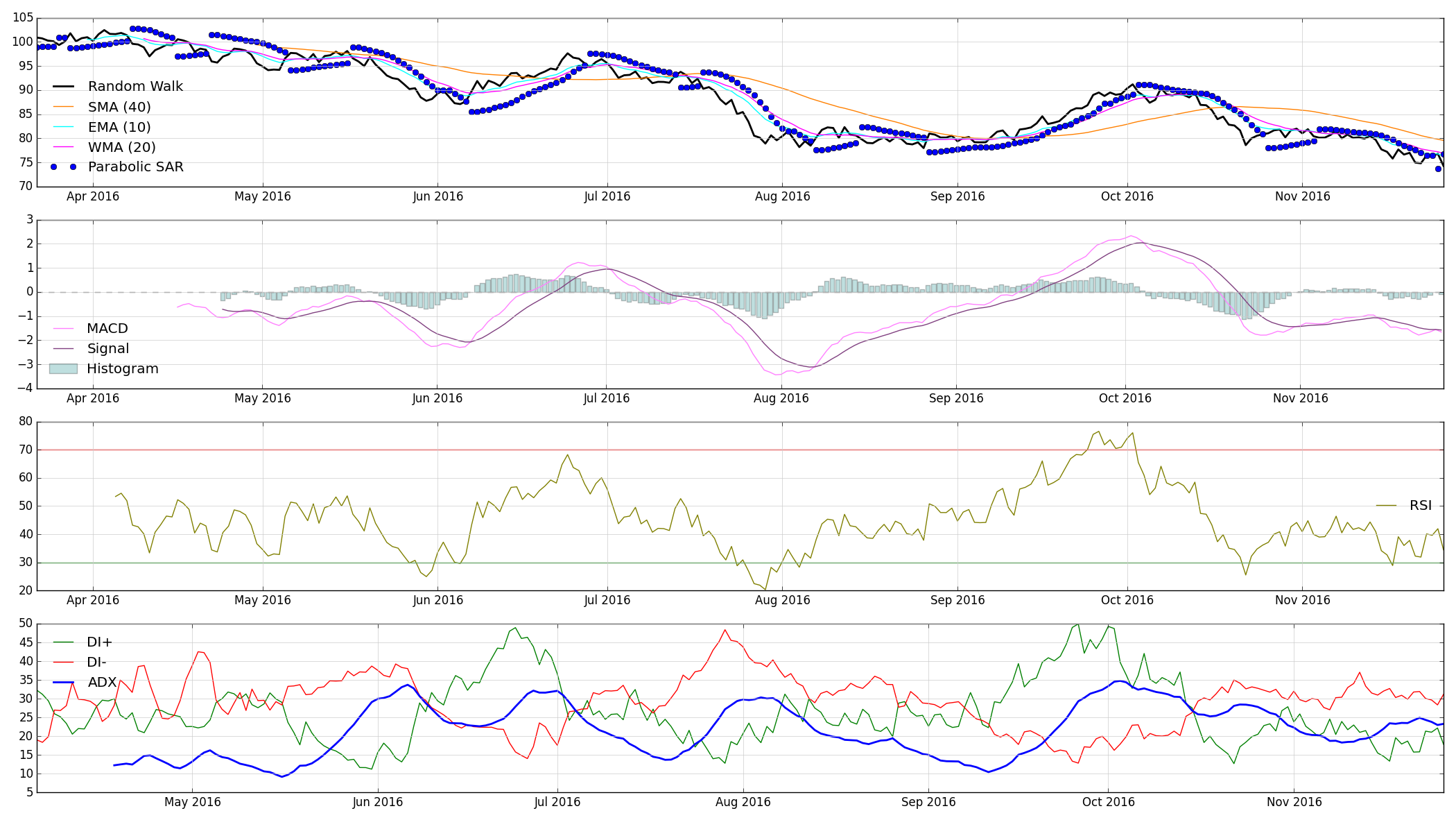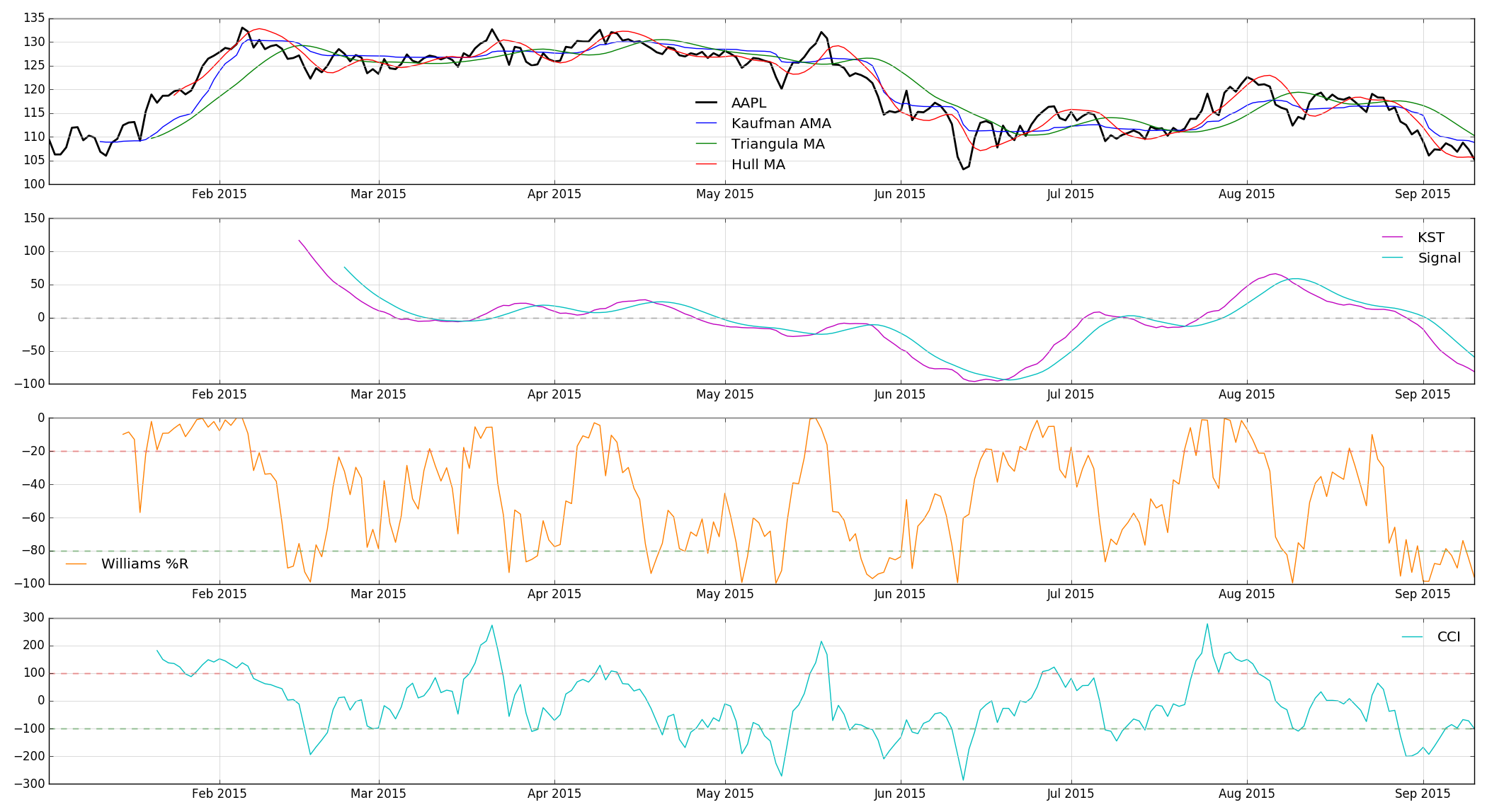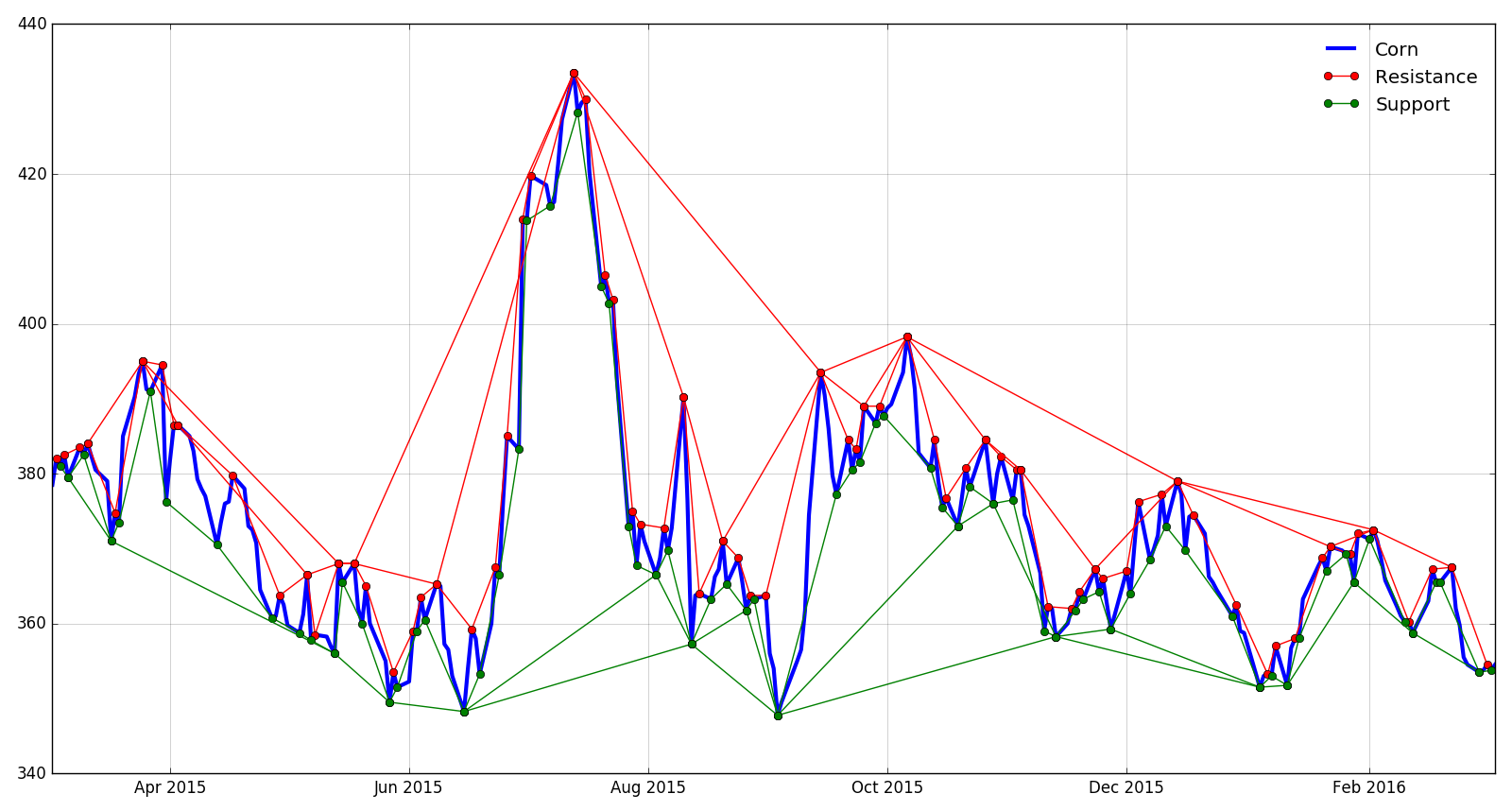# Indicators.jl

Financial market technical analysis & indicators in Julia# Indicators

Indicators is a Julia package offering efficient implementations of many technical analysis indicators and algorithms. This work is inspired by the TTR package in R and the Python implementation of TA-Lib, and the ultimate goal is to implement all of the functionality of these offerings (and more) in Julia.

## Implemented

### Moving Averages

• SMA (simple moving average)
• WMA (weighted moving average)
• EMA (exponential moving average)
• TRIMA (triangular moving average)
• KAMA (Kaufman adaptive moving average)
• MAMA (MESA adaptive moving average, developed by John Ehlers)
• HMA (Hull moving average)
• ALMA (Arnaud-Legoux moving average)
• SWMA (sine-weighted moving average)
• DEMA (double exponential moving average)
• TEMA (tripe exponential moving average)
• MLR (moving linear regression)
• Prediction
• Slope
• Intercept
• Standard error
• Upper & lower bound
• R-squared

### Momentum Indicators

• Momentum (n-day price change)
• ROC (rate of change)
• MACD (moving average convergence-divergence)
• RSI (relative strength index)
• Parabolic SAR (stop and reverse)
• Fast & slow stochastics
• SMI (stochastic momentum indicator)
• KST (Know Sure Thing)
• Williams %R
• CCI (commodity channel index)
• Donchian channel
• Aroon indicator + oscillator

### Volatility Indicators

• Bollinger Bands
• Average True Range
• Keltner Bands

### Other

• Rolling/running mean
• Rolling/running standard deviation
• Rolling/running variance
• Rolling/running covariance
• Rolling/running correlation
• Rolling/running maximum
• Rolling/running minimum
• Rolling/running MAD (mean absolute deviation)

## Todo

• Moving Linear Regression
• KAMA (Kaufman adaptive moving average)
• DEMA (double exponential moving average)
• TEMA (tripe exponential moving average)
• ALMA (Arnaud Legoux moving average)
• Parabolic SAR
• Williams %R
• KST (know sure thing)
• CCI (commodity channel index)
• ROC (rate of change)
• Momentum
• Donchian Channel
• Aroon Indicator / Aroon Oscillator
• Stochastics
• Slow Stochastics
• Fast Stochastics
• Stochastic Momentum Index
• Hamming moving average
• MMA (modified moving average)
• VWMA (volume-weighted moving average)
• VWAP (volume-weighted average price)
• ZLEMA (zero lag exponential moving average)
• EVWMA (elastic, volume-weighted moving average)
• VMA (variable-length moving average)
• Chaikin Money Flow
• Ultimate Oscillator
• OBV (on-balance volume)
• Too many more to name…

# Examples

#### Randomly generated data:#### Apple (AAPL) daily data from 2015:#### Corn futures daily data